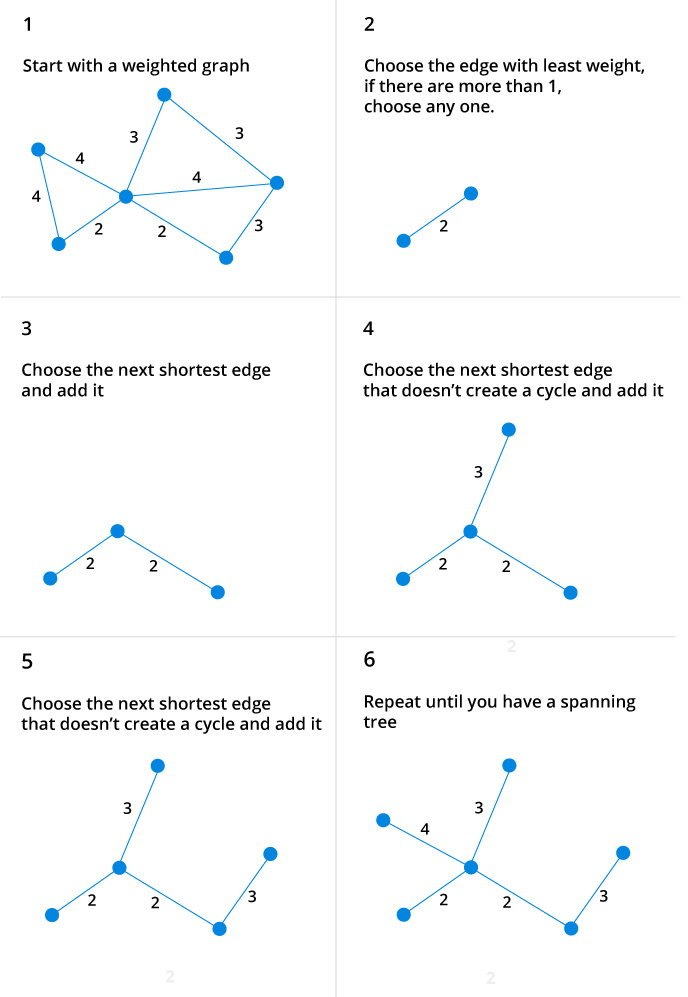Kruskal's Algorithm

# Kruskal's Algorithm

Kruskal's algorithm is a minimum spanning tree algorithm that takes a graph as input and finds the subset of the edges of that graph which

• form a tree that includes every vertex
• has the minimum sum of weights among all the trees that can be formed from the graph

## How Kruskal's algorithm works

It falls under a class of algorithms called greedy algorithms which find the local optimum in the hopes of finding a global optimum.

We start from the edges with the lowest weight and keep adding edges until we we reach our goal.

The steps for implementing Kruskal's algorithm are as follows:

1. Sort all the edges from low weight to high
2. Take the edge with the lowest weight and add it to the spanning tree. If adding the edge created a cycle, then reject this edge.
3. Keep adding edges until we reach all vertices.

## Example of Kruskal's algorithm## Kruskal Algorithm Pseudocode

Any minimum spanning tree algorithm revolves around checking if adding an edge creates a loop or not.

The most common way to find this out is an algorithm called Union FInd. The Union-Find algorithm divides the vertices into clusters and allows us to check if two vertices belong to the same cluster or not and hence decide whether adding an edge creates a cycle.

``````KRUSKAL(G):
A = ∅
For each vertex v ∈ G.V:
MAKE-SET(v)
For each edge (u, v) ∈ G.E ordered by increasing order by weight(u, v):
if FIND-SET(u) ≠ FIND-SET(v):
A = A ∪ {(u, v)}
UNION(u, v)
return A
``````

## Kruskal's Algorithm Implementation in C++

Here is the code for C++ implementation in C++. We use standard template libraries to make our work easier and code cleaner.

``````#include <iostream>
#include <vector>
#include <algorithm>
using namespace std;

#define edge pair<int,int>

class Graph {
private:
vector<pair<int, edge>> G; // graph
vector<pair<int, edge>> T; // mst
int *parent;
int V; // number of vertices/nodes in graph
public:
Graph(int V);
void AddWeightedEdge(int u, int v, int w);
int find_set(int i);
void union_set(int u, int v);
void kruskal();
void print();
};
Graph::Graph(int V) {
parent = new int[V];

//i 0 1 2 3 4 5
//parent[i] 0 1 2 3 4 5
for (int i = 0; i < V; i++)
parent[i] = i;

G.clear();
T.clear();
}
void Graph::AddWeightedEdge(int u, int v, int w) {
G.push_back(make_pair(w, edge(u, v)));
}
int Graph::find_set(int i) {
// If i is the parent of itself
if (i == parent[i])
return i;
else
// Else if i is not the parent of itself
// Then i is not the representative of his set,
// so we recursively call Find on its parent
return find_set(parent[i]);
}

void Graph::union_set(int u, int v) {
parent[u] = parent[v];
}
void Graph::kruskal() {
int i, uRep, vRep;
sort(G.begin(), G.end()); // increasing weight
for (i = 0; i < G.size(); i++) {
uRep = find_set(G[i].second.first);
vRep = find_set(G[i].second.second);
if (uRep != vRep) {
union_set(uRep, vRep);
}
}
}
void Graph::print() {
cout << "Edge :" << " Weight" << endl;
for (int i = 0; i < T.size(); i++) {
cout << T[i].second.first << " - " << T[i].second.second << " : "
<< T[i].first;
cout << endl;
}
}
int main() {
Graph g(6);
g.kruskal();
g.print();
return 0;
}``````

When we run the program, we get output as

```Edge : Weight
1 - 2 : 2
2 - 5 : 2
2 - 3 : 3
3 - 4 : 3
0 - 1 : 4```

## Kruskal's vs Prim's Algorithm

Prim's algorithm is another popular minimum spanning tree algorithm that uses a different logic to find the MST of a graph. Instead of starting from an edge, Prim's algorithm starts from a vertex and keeps adding lowest-weight edges which aren't in the tree, until all vertices have been covered.